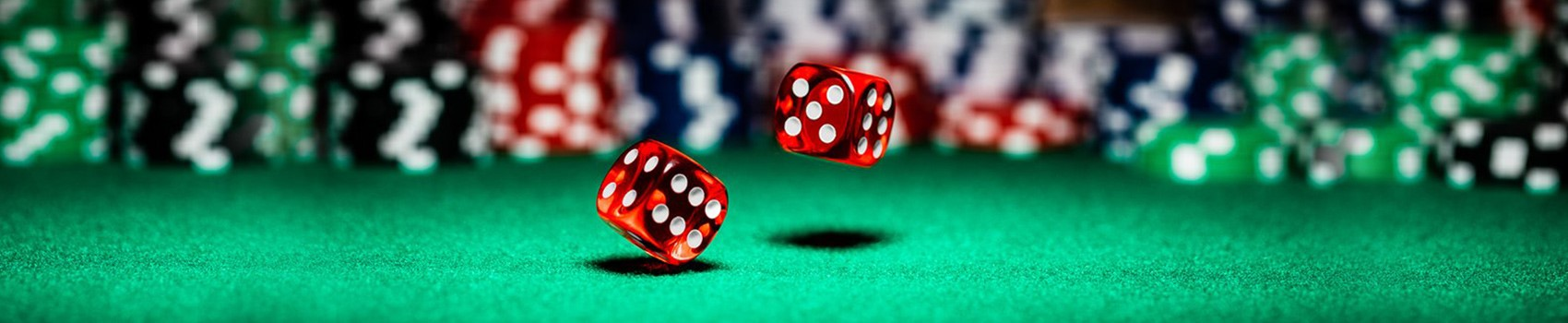# Golden Touch Craps

Probability or Odds --- Same or Different?

People sometimes use these terms interchangeably for the same mathematical expression. Other times they use one term when they should be using the other. In the big scheme of things this is not all that significant. One need not get too concerned if one uses the wrong term because folks will often understand what you mean either way.

I thought it would be helpful to explain the difference between the two terms for those who may be unclear about when to use one versus the other and would like to be better informed about the proper meaning of the two terms.

OK, let's get probability and odds squared away. Essentially they are expressing the same thing but doing them in a slightly different manner. Odds are expressed as a relationship between an event occurring or not and probabilities are expressed as a ratio of possible events to total events. Probabilities by definition are therefore between 0 and 1 inclusively. They are commonly expressed as a fraction, decimal or percentage.

All right, I know that is "math speak" and I may have lost some of you with it. Please bear with me and I hope it will become clearer.

In other words if we look at an event that can occur,

The odds of it occurring are expressed as the relation of the chance FOR it occurring to the chances AGAINST it occurring.

Odds = (Chances for):(Chances against)

The probability of it occurring is expressed as the ratio of the chances FOR it occurring to the TOTAL chances that can occur (the sum of those for and against).

Probability = (Chances for)/(Total Chances) where Total Chances equals the sum of Chances for and Chances against.

For example, getting a winning bet on the Iron Cross. The Iron Cross is a wager where one makes a field bet in addition to a place bet on the 5, 6 and 8. You will lose all four of your wagers if a seven is thrown and win at least one of your bets if any other number is thrown. There are 36 possible ways for the dice to land. There are 6 ways to throw a seven and 30 ways to throw one of the other numbers. You need to throw any number other than a seven to win one of your bets.

The odds are 30:6 or 30 to 6, which could be reduced to 5:1, or 5 to 1.

The probability of winning a bet with the Iron Cross would be 30/36, 5/6, .833 or 83%.

There is no conjecture or guessing in calculating the probability. It has the same degree of accuracy as the odds.

The odds of throwing a head with a single flip of a coin are 1:1 or 1 to 1. The probability of a head is 1/2, .5 or 50%. The odds and probability are both taking the same data into consideration. They are both looking at the possibility of 1 event occurring out of 2 possible outcomes.

Yeah, I know. I promised it would become clearer and your eyes are glazing over at this point. But I will make a wager with you that you understand this much better than you think you do. Try the following example and see if you get the proper answer.

I have a box with 4 black balls and 1 white ball, all the same size and weight, inside it. You select 1 ball at random from inside the box.

What is the probability you will select the white ball?

Probability = (Chances for)/(Total Chances) where Total Chances equals the sum of Chances for and Chances against.

What are the odds you will select the white ball?

Odds = (Chances for):(Chances against)

Stop here and try to figure it out before reading any further.

You have to answer both questions.

Did you get the right answers?

I made a wager with you on this. We are betting even money on the outcome. But I am making this wager with all the people who want to make the bet. I am making this bet with a number of different people.

I only win money if more people who take the wager get the right answer than the wrong answer. I do not expect everyone to get the right answer. But if a smaller number get the wrong answer than the right answer I will win money.

I think the probability of getting the wrong answer is 1/5, .20 or 20%.

I think the odds of getting the wrong answer are 1:4 or 1 to 4.

And those are the same answers you should have gotten for the problem I gave you above.

How did I do with my wager?